# Dendro-5.01## What is Dendro ?

“Dendro” in Greek language means tree. The Dendro library is a large scale (262K cores on ORNL’s Titan) distributed memory adaptive octree framework. The main goal of Dendro is to perform large scale multiphysics simulations efficeiently in mordern supercomputers. Dendro consists of efficient parallel data structures and algorithms to perform variational ( finite element) methods and finite difference mthods on 2:1 balanced arbitary adaptive octrees which enables the users to perform simulations raning from black holes (binary black hole mergers) to blood flow in human body, where applications ranging from relativity, astrophysics to biomedical engineering.

## Get Dendro (git repo )

You can clone the repository using , `git clone https://github.com/paralab/Dendro-5.01.git`

## Dendro-5.01 Documentation

Doxygen documentation can be found here

## How to build Dendro-5.0 ?

You need CMake to build dendro. Create a build directory using ‘mkdir build’. Then go into the build directory by ‘cd build’ then execute ‘ccmake ..’ to generate the make files. You can build Dendro-5.0 with several options.

• `ALLTOALLV_FIX` : OFF,Need to turn off
• `DIM_2`: OFF, This can be turned on if you need to run Dendro-5.0 in 2D case. default: OFF (Which means it assumes 3D domain)
• `HILBERT_ORDERING`:ON, This specify which SFC to use to partition the data. HILBERT_ORDERING: ON means it uses Hilbert curve, otherwise it uses Morton curve for partitioning.
• `PROFILE_TREE_SORT`: OFF
• `NUM_NPES_THRESHOLD`: square root of P (number of processors)
• `SPLITTER_SELECTION_FIX`: ON. This will perform the data exchange in the octree partitioning in stages. This is mandatory when you run dendro in very large scale.

## Simple simulation: Nonlinear Sigma Model (NLSigma)

NlSigma folder consists of simple, non lineat wave equation with adaptive mesh refinement (AMR). You can copy the parameter file from `NLSigma/par` folder and simply run `mpirun -np 8 ./NLSigma/nlsmSolver nlsm.par.json`, on your lattop to large supercomputer with higher resolution.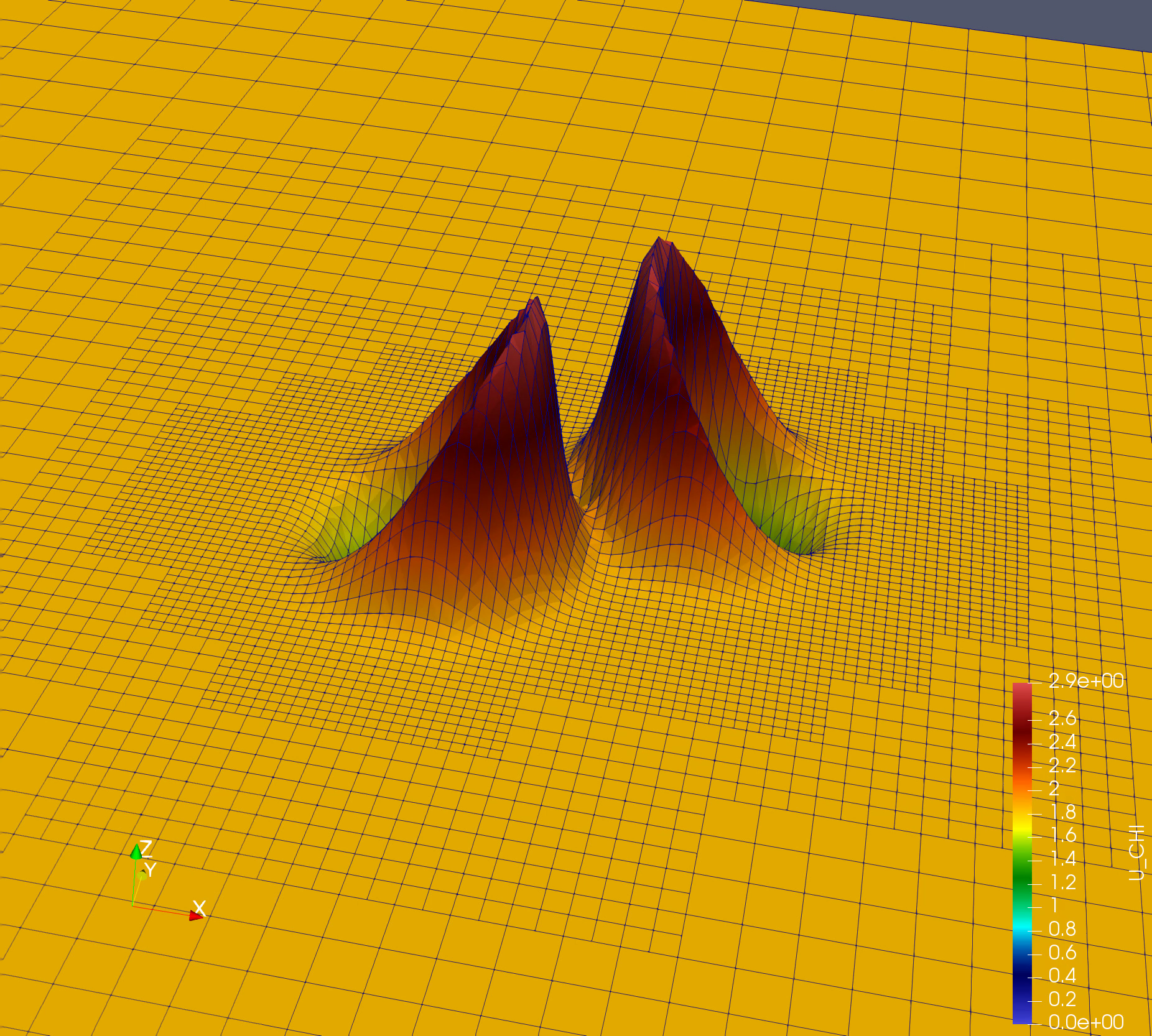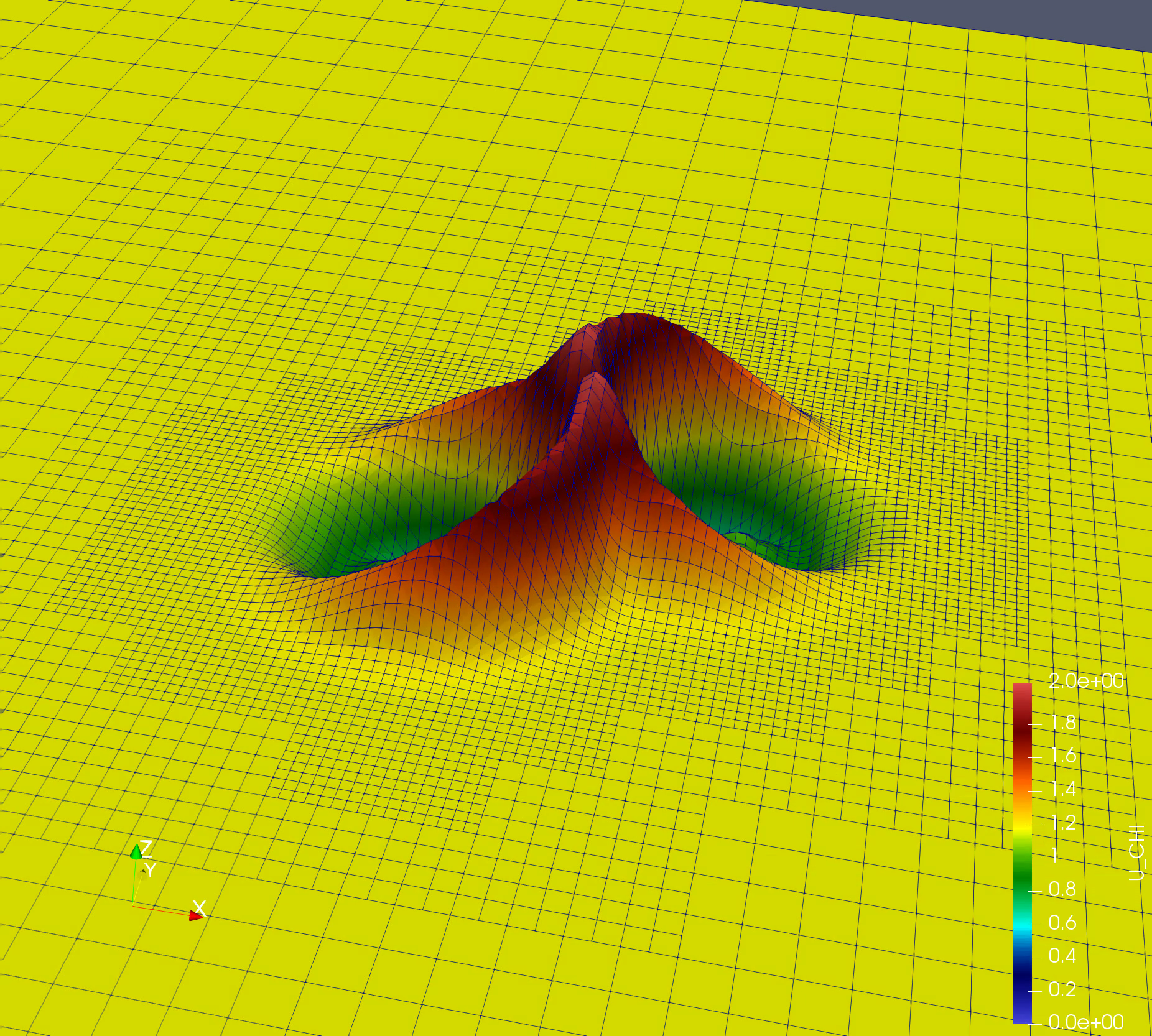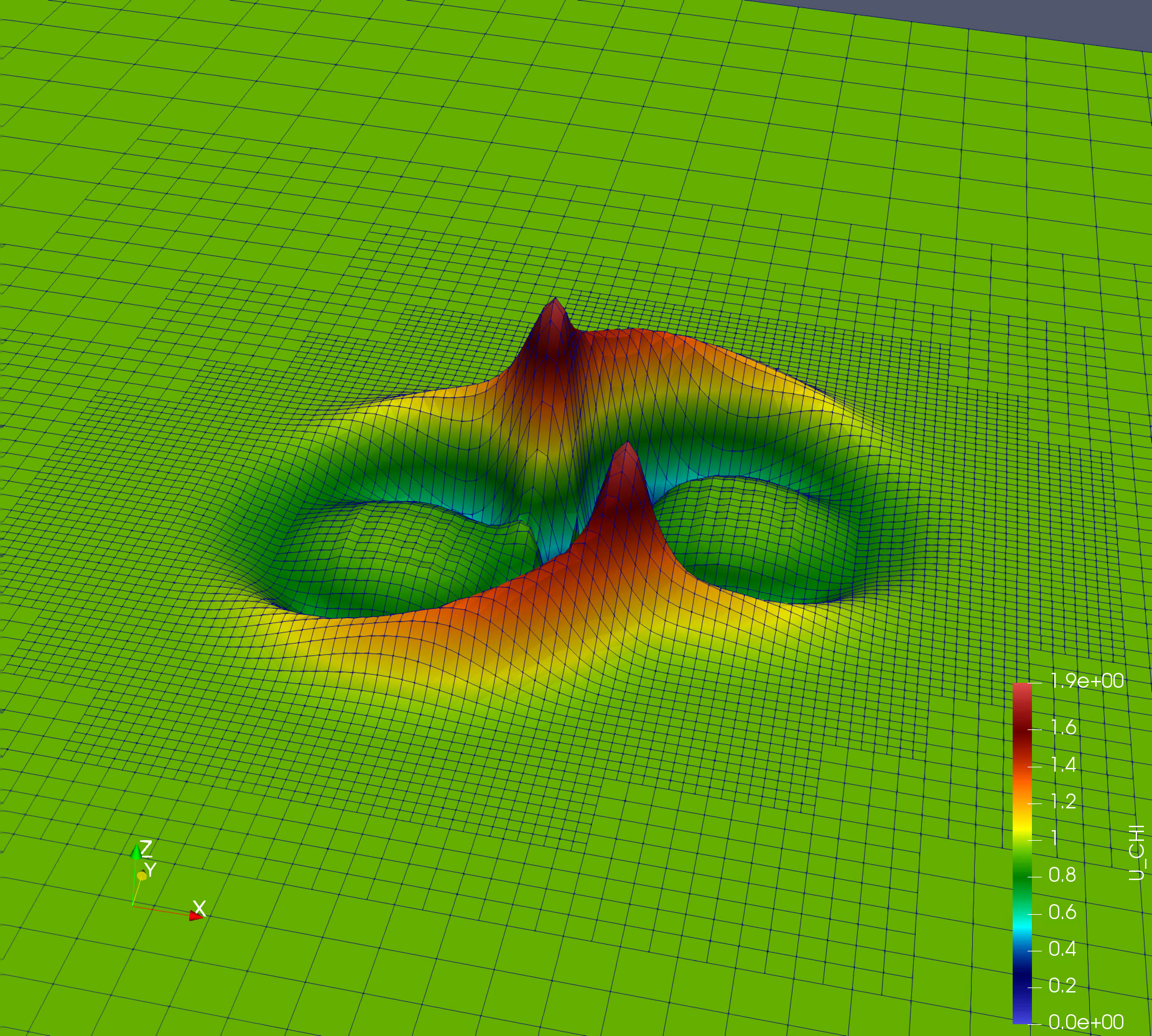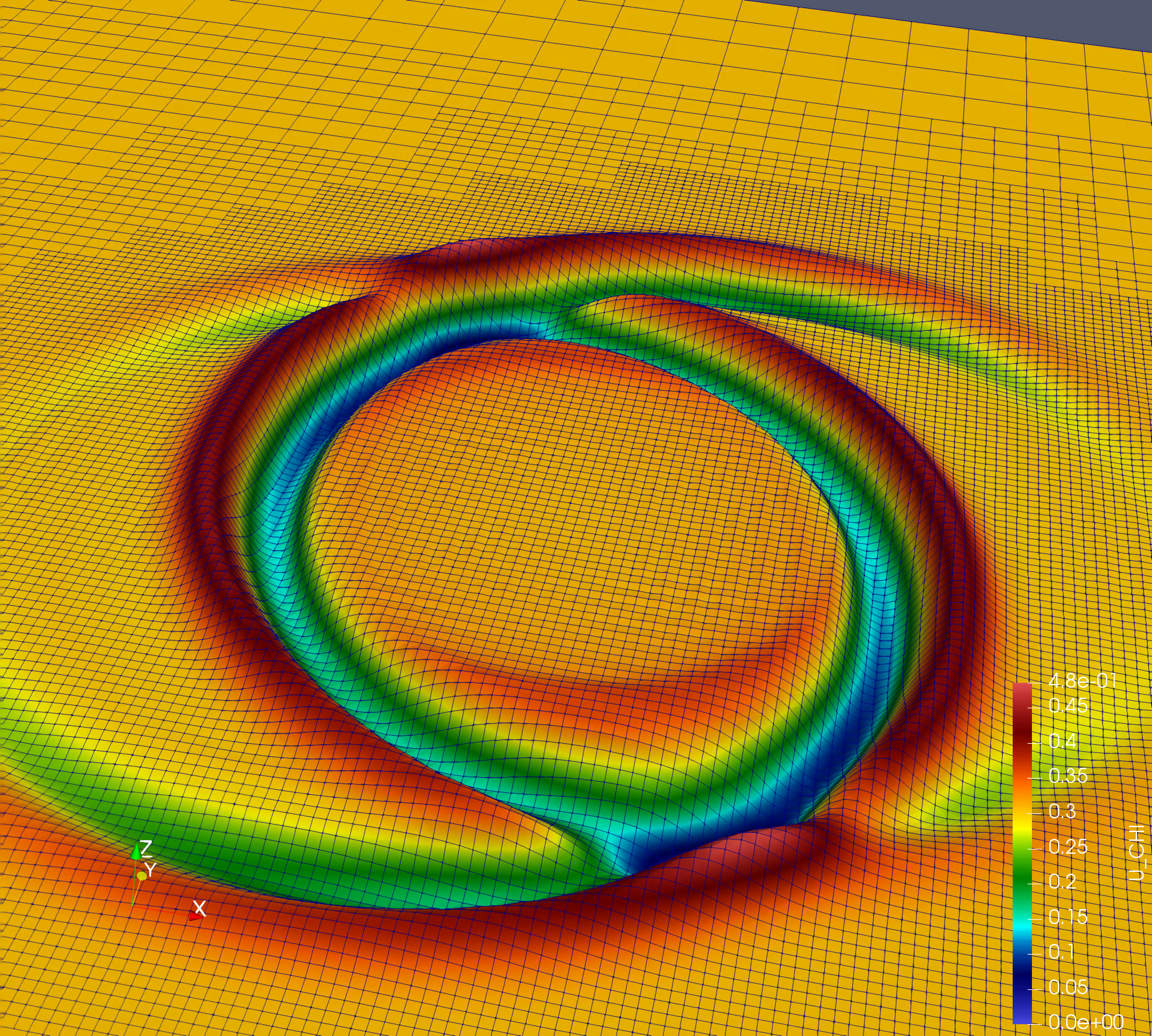You can write the equations in symbolic python which generate the C compute kernel. Look at `nlsm.py`

``````import dendro
from sympy import *
###############################################################
#  initialize
###############################################################
r = symbols('r')
# declare functions
chi = dendro.scalar("chi","[pp]")
phi = dendro.scalar("phi","[pp]")
d = dendro.set_first_derivative('grad')    # first argument is direction
d2s = dendro.set_second_derivative('grad2')  # first 2 arguments are directions
d2 = dendro.d2

###############################################################
#  evolution equations
###############################################################

phi_rhs = sum( d2(i,i,chi)  for i in dendro.e_i ) - sin(2*chi)/r**2
chi_rhs = phi

###############################################################
#  evolution equations
###############################################################
outs = [phi_rhs, chi_rhs]
vnames = ['phi_rhs', 'chi_rhs']
dendro.generate(outs, vnames, '[pp]')
``````

Which generate the code,

``````// Dendro: original ops:  10
// Dendro: printing temp variables
// Dendro: printing variables
//--
//--
chi_rhs[pp] = phi[pp];
// Dendro: reduced ops:  10
``````

### Parameters for NLSigma

• Grid parameters
• `NLSM_GRID_MIN_X`, `NLSM_GRID_MIN_Y`, `NLSM_GRID_MIN_Z`: The minimum coordinate values for the computational domain in the x-, y-, and z- directions.
• `NLSM_GRID_MAX_X`, `NLSM_GRID_MAX_Y`, `NLSM_GRID_MAX_Z`: The maximum coordinate values for the computational domain in the x-, y-, and z- directions.
• Evolution parameters
• `NLSM_CFL_FACTOR`: The Courant factor used for time integration. dt = `NLSM_CFL_FACTOR *` dx
• `KO_DISS_SIGMA`: Coefficient for Kreiss-Oliger dissipation that is added to the solution.
• `NLSM_RK45_TIME_BEGIN`: Initial time label for the evolution, usually this is set to 0.
• `NLSM_RK45_TIME_END`: The final time for the evolution. The code exits when this time is reached.
• `NLSM_RK45_TIME_STEP_SIZE`: Initial time step for the Runge-Kutta 4-5 adaptive time integrator.
• `NLSM_RK45_DESIRED_TOL`: Tolerance for the RK4-5 adaptive time integrator.
• Output parameters
• `NLSM_IO_OUTPUT_FREQ`: Frequency for output. Output is written as 3D VTK files.
• `NLSM_VTU_FILE_PREFIX`: Prefix for naming the output files. Each processor outputs all variables to a single file labeled by the timestep.
• `NLSM_NUM_EVOL_VARS_VTU_OUTPUT`: The number of evolution variables to be output.
• `NLSM_VTU_OUTPUT_EVOL_INDICES`: A list of variable indices to specify which variables will be written to output. The first `NLSM_NUM_EVOL_VARS_VTU_OUTPUT` in this list are written to the output file.
• General refinement parameters
• `NLSM_MAXDEPTH`: The maximum refinement depth for the octree. The minimum possible grid resolution is proportional to 1/2^k, where k is the maximum depth.
• `NLSM_REMESH_TEST_FREQ`: Frequency for updating an adaptive grid.
• `NLSM_LOAD_IMB_TOL`: Dendro load imbalance tolerance for flexible partitioning.
• `NLSM_DENDRO_GRAIN_SZ`: Grain size N/p , Where N number of total octants, p number of active cores. This is essentially the number of octants per core, and essentially functions as a measure of the computational load per core. (Dendro does not automatically use all cores that are available in a multiprocessor run, but gives each core a minimum amount of work before adding new cores.)
• Wavelet refinement parameters
• `NLSM_WAVELET_TOL`: The wavelet error tolerance for the solution. Refinement is added when the estimated error in the solution, measured by the wavelet coefficient, is larger than this tolerance.
• `NLSM_NUM_REFINE_VARS`: The number of variables used to evaluate refinement on the grid.
• `NLSM_REFINE_VARIABLE_INDICES`: A list of variable indicies to specify which variables are used to determine the grid refinement. Wavelet coefficients will be calculated for the first `NLSM_NUM_REFINE_VARS` in this list.
• `NLSM_DENDRO_AMR_FAC`: A factor to determine the threshold for coarsening the grid. The grid is coarsened when the wavelet coefficients are less than `NLSM_DENDRO_AMR_FAC * NLSM_WAVELET_TOL`.
• Block refinement parameters
• `NLSM_ENABLE_BLOCK_ADAPTIVITY`: Block adaptivity provides simple fixed-mesh refinement. The grid is refined to the maximum depth (or minimum resolution) inside a fixed region set by `NLSM_BLK_MIN_X`, `NLSM_BLK_MAX_X`, and related variables. Set this parameter to “1” to enable block adaptivity, or “0” otherwise (default). Block adaptivity is used primarily for testing and debugging. For example, a uniform grid can be created by specifying maximum refinement region to cover the entire domain.
• `NLSM_BLK_MIN_X`: The minimum x-coordinate for the block that is refined to the maximum depth. Same for y- and z-coordinates.
• `NLSM_BLK_MAX_X`: The maximum x-coordinate for the block that is refined to the maximum depth. Same for y- and z-coordinates.
• Checkpoint parameters
• `NLSM_RESTORE_SOLVER`: Set this parameter to “1” to read from checkpoint files, otherwise set it to “0” (default). When checkpointing is used, the code automatically selects the latest checkpoint files for restoring the solver.
• `NLSM_CHECKPT_FREQ`: The checkpoint frequency.
• `NLSM_CHKPT_FILE_PREFIX`: A string prefix for naming the checkpoint files.

## Scalability on octree generation and partitioning.

We have performed octree generation and partitioning up to 262144 cores in ORNL’s titan super computer. We have managed to partition 1.3x10^12 octants among 262144 processors with in 4 seconds.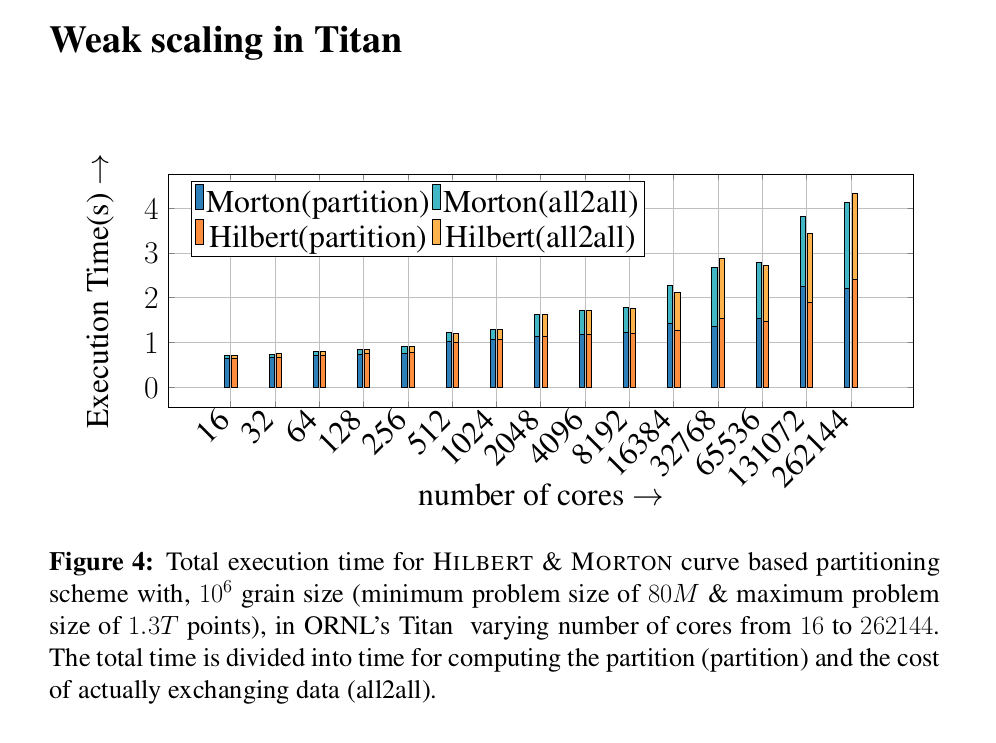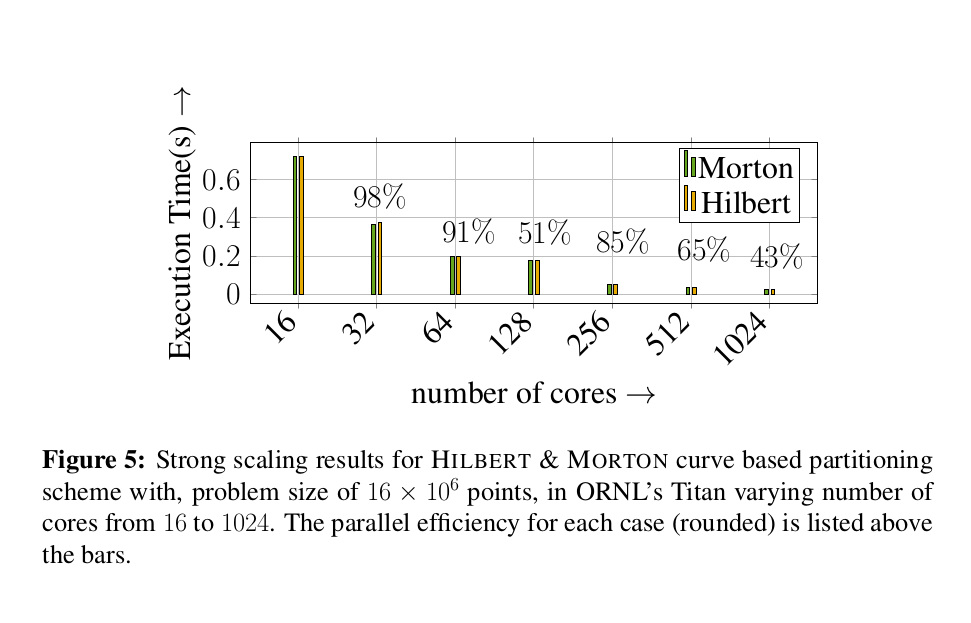## Publications

• Milinda Fernando, David Neilsen, Hyun Lim, Eric Hirschmann, Hari Sundar, ”Massively Parallel Simulations of Binary Black Hole Intermediate-Mass-Ratio Inspirals” SIAM Journal on Scientific Computing 2019. ‘https://doi.org/10.1137/18M1196972’
• Milinda Fernando, David Neilsen, Hari Sundar, ”A scalable framework for Adaptive Computational General Relativity on Heterogeneous Clusters”, (ACM International Conference on Supercomputing, ICS’19)
• Milinda Fernando, Dmitry Duplyakin, and Hari Sundar. 2017. ”Machine and Application Aware Partitioning for Adaptive Mesh Refinement Applications”. In Proceedings of the 26th International Symposium on High-Performance Parallel and Distributed Computing (HPDC ’17). ACM, New York, NY, USA, 231-242. DOI: ‘https://doi.org/10.1145/3078597.3078610’